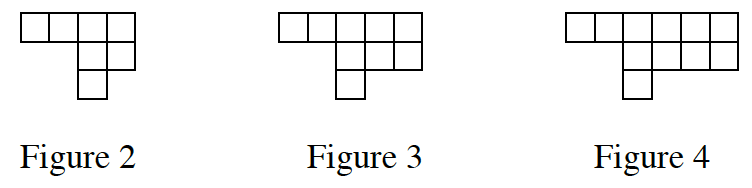### Home > CC4 > Chapter 2 > Lesson 2.2.2 > Problem2-61

2-61.

Write the equation for the following tile pattern. Homework Help ✎Make a table of data, relating the figure number (as $x$), and the number of squares $(y)$.